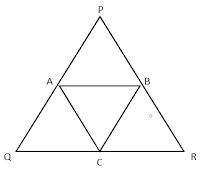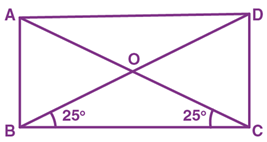### Maths MCQ Class IX Ch-14 | Statistics

Mathematics  MCQ | Class 09 | Chapter 14 STATISTICS Multiple Choice Questions (MCQ) MCQ Based on the Data. MCQ  Based on the Mean of Data. MCQ Based on the Median. MCQ Based on the Mode. Features In the MCQ given below you find the important MCQ which are strictly according to the CBSE syllabus and are very useful for the CBSE Examinations.  Solution Hints are also given to some difficult problems.  Each MCQ contains four options from which one option is correct.  Action Plan First of all students should Learn and write all basic points and Formulas related to the Chapter 14 Statistics. Start solving  the NCERT Problems with examples. Solve the important assignments on the Chapter 14 Class IX. Then start solving the following MCQ. MCQ |Chapter 14 | Statistics | Class IX

Mathematics

## MCQ Based on the differentiating different types of quadrilaterals.

### Features

• In the MCQ given below you find the important MCQ which are strictly according to the CBSE syllabus and are very useful for the CBSE Examinations.
• Solution Hints are also given to some difficult problems.
• Each MCQ contains four options from which one option is correct.

### Action Plan

• First of all students should Learn and write all basic points and Formulas related to the Chapter 8 Quadrilaterals.
• Start solving  the NCERT Problems with examples.
• Solve the important assignments on the Chapter 8 Class XI.
• Then start solving the following MCQ.

# MCQ | CHAPTER - 8 | CLASS IXQUADRILATERAL

Q1) The quadrilateral whose all its sides are equal and angles are equal to 90 degrees, it is called:

a) Rectangle

b) Square

c) Kite

d) Parallelogram

a) one
b) two
c) three
d) four

Q3) The sum of all the angles of a quadrilateral is equal to:

a) 180°

b) 270°

c) 360°

d) 90°

Q4) The sum of four angles of quadrilateral is equal to _______
a) 90°
b) 360°
c) 180°
d) 270°

Q5) A trapezium has:

a) One pair of opposite sides parallel

b) Two pairs of opposite sides parallel to each other

c) All its sides are equal

d) All angles are equal

Q7) A rhombus can be a:

a) Parallelogram

b) Trapezium

c) Kite

d) Square

Q8) At which angle do diagonals of a rhombus bisects each other?
a) 180°
b) 360°
c) 270°
d) 90°

Q9) A diagonal of a parallelogram divides it into two congruent:

a) Square

b) Parallelogram

c) Triangles

d) Rectangle

Q10) What is the quadrilateral formed by the angle bisectors of a parallelogram?
a) Square
b) Rectangle
c) Circle
d) Rhombus

Q11) In a parallelogram, opposite angles are:

a) Equal

b) Unequal

c) Cannot be determined

d) None of the above

Q12) Find the value of x if PQRS is a parallelogram.

Q13) The diagonals of a parallelogram are

a) Equal

b) Unequal

c) Bisect each other

d) Have no relation

Q14) From the figure find the length of AD if perimeter of parallelogram ABCD is 26cm.

a) 10 cm
b) 15 cm
c) 12 cm
d) 5 cm

Q15) Each angle of the rectangle is:

a) More than 90°

b) Less than 90°

c) Equal to 90°

d) Equal to 45°

Q16) The angles of a quadrilateral are in the ratio 4 : 5 : 10 : 11. The angles are:

a) 36°, 60°, 108°, 156°

b) 48°, 60°, 120°, 132°

c) 52°, 60°, 122°, 126°

d) 60°, 60°, 120°, 120°

Explanation:

As per angle sum property, we know:

4x+5x+10x+11x = 360°

30x = 360°

x = 12°

Hence, angles are

4x = 4 (12) = 48°

5x = 5 (12) = 60°

10x = 10 (12) = 120°

11x = 11 (12) = 132°

Q17) If ABCD is a trapezium in which AB || CD and AD = BC, then:

a) A = B

b) A > B

c) A < B

d) None of the above

Explanation : See Q12 Exercise 8.1 NCERT

Q18) Which of the following is not true for a parallelogram?

a) Opposite sides are equal

b) Opposite angles are equal

c) Opposite angles are bisected by the diagonals

d) Diagonals bisect each other.

Q19) If the ratio of four angles of a quadrilateral is A : B : C : D = 1 : 2 : 3 : 4, what is the value of C?
a) 90°
b) 108°
c) 180°
d) 72°

Q20) Three angles of a quadrilateral are 75º, 90º and 75º. The fourth angle is

a) 90º

b) 95º

c) 105º

d) 120º

Explanation: We know that the sum of angles of a quadrilateral is 360º.

Let the unknown angle be x.

Therefore, 75º + 90º + 75º + x = 360º

x = 360º – 240º = 120º.

Q21) What is the length of DE if DE || BC and D and E are midpoints of AB and AC?

a) 18cm
b) 15cm
c) 9cm
d) 20cm

Q22) ABCD is a rhombus such that ACB = 40º. Then ADB is

(a) 40º

(b) 45º

(c) 50º

(d) 60º

Explanation: We know that the diagonals of the rhombus bisect each other perpendicularly.

By using the alternate interior angles, and angle sum property of triangle, we can say:

From the triangle, BOC,

BOC + OCB + OBC = 180º

(where  BOC= 90º, OCB = 40º)

90º + 40º + OBC = 180º

OBC = 180º – 130º

OBC = 50º

OBC =DBC

Now, by using alternate angles, we can say

Q23)  Find the perimeter of ΔABC, if perimeter of ΔPQR is 36cm and A, B and C are midpoints.a) 9cm
b) 18cm
c) 20cm
d) 36cm

Q24) The quadrilateral formed by joining the mid-points of the sides of a quadrilateral PQRS, taken in order, is a rhombus, if

a) PQRS is a rhombus

b) PQRS is a parallelogram

c) Diagonals of PQRS are perpendicular

d) Diagonals of PQRS are equal.

Explanation: The quadrilateral formed by joining the mid-points of the sides of a quadrilateral PQRS, taken in order, is a rhombus if the diagonals of PQRS are equal.

Q25) A diagonal of a parallelogram divides it into two congruent:
a. Square
b. Parallelogram
c. Triangles
d. Rectangle

Q26) A diagonal of a rectangle is inclined to one side of the rectangle at 25º. The acute angle between the diagonals isa) 25º

b) 40º

c) 50º

d) 55º

Explanation: Consider the rectangle ABCD

In a triangle BOC,

OBC = OCB (Opposite angles of isosceles triangle)

Therefore, OBC + OCB + BOC = 180º

25º + 25º + BOC = 180º

BOC = 180º- 50º

BOC = 130º.

By using the linear pair,

AOB + BOC = 180º

AOB = 180º – 130º

AOB= 50º

Hence, the acute angle between the diagonals is 50º.

Q27) In a parallelogram, opposite angles are:
a. Equal
b. Unequal
c. Cannot be determined
d. None of the above

Q28) If angles A, B, C and D of the quadrilateral ABCD, taken in order, are in the ratio 3 : 7 : 6 : 4, then ABCD is a

a) Kite

b) Rhombus

c) Parallelogram

d) Trapezium

Explanation: Given that, the angles A, B, C and D of a quadrilateral are in the ratio 3 : 7 : 6 : 4.

We know that A + B + C + D = 360º

Hence, now we can assume, 3x + 7x + 6x + 4x = 360º

20x = 360º

x=18º

Therefore, A = 3x = 54º

B = 7x = 126º

C = 6x = 108º

D = 4x= 72º

Here A + B = 180o

And C + D = 180o

ABCD is a quadrilateral with one pair of opposite sides are parallel.

ABCD is a trapezium

Q29) The quadrilateral formed by joining the mid-points of the sides of a quadrilateral PQRS, taken in order, is a rectangle, if

a) PQRS is a rectangle

b) PQRS is a parallelogram

c) Diagonals of PQRS are perpendicular

d) Diagonals of PQRS are equal

Explanation: The quadrilateral formed by joining the mid-points of the sides of a quadrilateral PQRS, taken in order, is a rectangle, if the diagonals of PQRS are perpendicular.

Q30) Each angle of rectangle is:
a. More than 90°
b. Less than 90°
c. Equal to 90°
d. Equal to 45°

Q31) The figure obtained by joining the mid-points of the sides of a rhombus, taken in order, is

a) a square

b) a rhombus

c) a rectangle

d) any parallelogram

Explanation: See Q2 NCERT Ex- 8.2

Let ABCD be a rhombus. P, Q, R, S be the midpoint of the sides AB, BC, CD and DA. If we join the midpoints, we will get the shape rectangle.

Q32) If APB and CQD are two parallel lines, then the bisectors of the angles APQ, BPQ, CQP and PQD form

a) Square

b) Rectangle

c) Rhombus

d) any other parallelogram

Explanation: Hence, the bisectors of the angles APQ, BPQ, CQP and PQD form the shape rectangle.

Q33) Which of the following is not a quadrilateral?

a) Kite

b) Square

c) Triangle

d) Rhombus